# Network Design under Demand Uncertainty Koonlachat Meesublak National

• Slides: 28Network Design under Demand Uncertainty Koonlachat Meesublak National Electronics and Computer Technology Center Thailand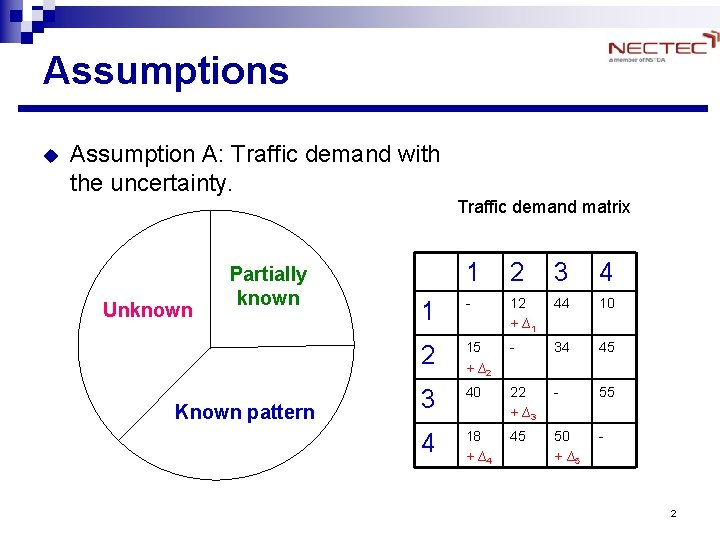Assumptions u Assumption A: Traffic demand with the uncertainty. Traffic demand matrix Unknown Partially known Known pattern 1 2 3 4 1 - 12 + ∆1 44 10 2 15 + ∆2 - 34 45 3 40 22 + ∆3 - 55 4 18 + ∆4 45 50 + ∆5 - 2Possible Approaches u How to handle the uncertainty? Bandwidth reservation approaches mean-rate based peak-rate based “average cases” “worst case” Pro: cheap design Pro: could handle large variation Con: rejection of demand Con: the most expensive design requests (large safety margin) Statistical approach ? 3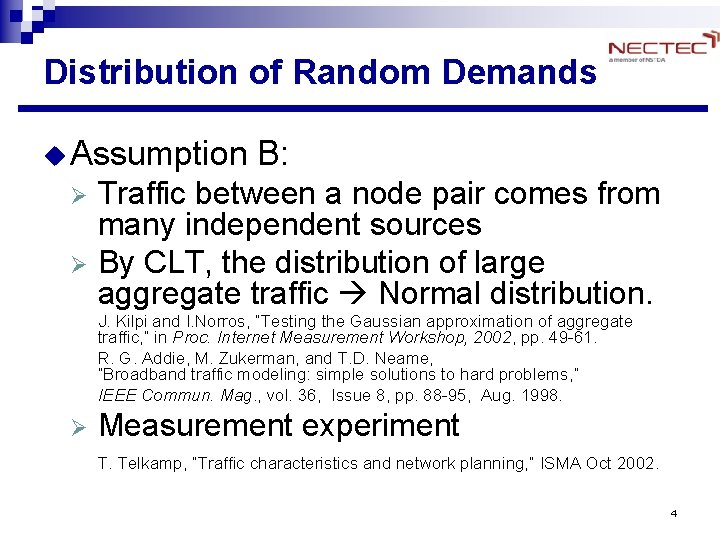Distribution of Random Demands u Assumption B: Traffic between a node pair comes from many independent sources Ø By CLT, the distribution of large aggregate traffic Normal distribution. Ø J. Kilpi and I. Norros, “Testing the Gaussian approximation of aggregate traffic, ” in Proc. Internet Measurement Workshop, 2002, pp. 49 -61. R. G. Addie, M. Zukerman, and T. D. Neame, “Broadband traffic modeling: simple solutions to hard problems, ” IEEE Commun. Mag. , vol. 36, Issue 8, pp. 88 -95, Aug. 1998. Ø Measurement experiment T. Telkamp, “Traffic characteristics and network planning, ” ISMA Oct 2002. 4Assumption B u u Thus, the traffic from large aggregation is not totally uncharacterized. Traffic distribution could be useful. Bandwidth reservation approaches mean-rate based “average case” Pro: cheap design Con: rejection of demand requests peak-rate based “worst case” Pro: could handle large variation Con: the most expensive design Based on demand ~ N (m, s 2) Benefits? How to deal with such demand? 5Benefits u Why use statistical allocation? Ø Bandwidth is not unlimited, and is not free consider the tradeoffs between cost and ability to handle the variation. These are the benefits between mean and peak schemes. How can we handle the uncertainty? E. g. , using m + 3 s can cover 99. 9% of the area. m s xs peak 6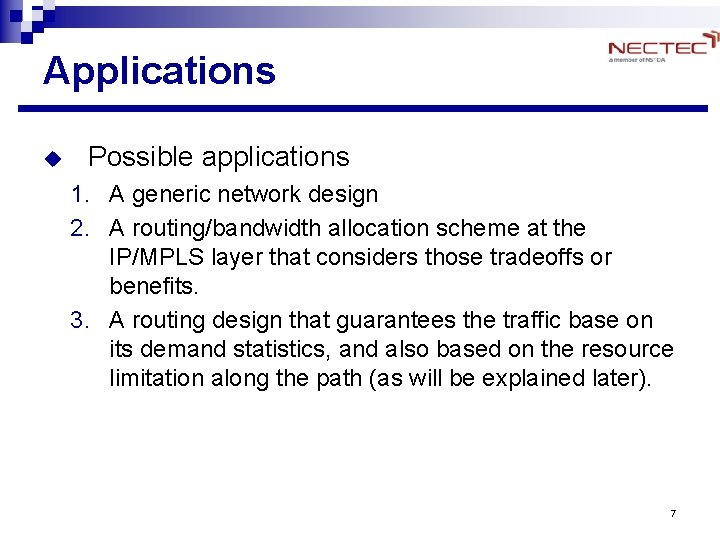Applications u Possible applications 1. A generic network design 2. A routing/bandwidth allocation scheme at the IP/MPLS layer that considers those tradeoffs or benefits. 3. A routing design that guarantees the traffic base on its demand statistics, and also based on the resource limitation along the path (as will be explained later). 7Related optimization models u Deterministic model Ø Demand is known or easily estimated l l Uses mean value or worst-case value Extension: time dimension, e. g. , multi-hour design. u Stochastic model Ø Ø Demand can be treated as a random variable Typical Long-term / multi-period planning design: l l l Stochastic Programming with Recourse ,  Robust Optimization  involves forecasting of future events. 8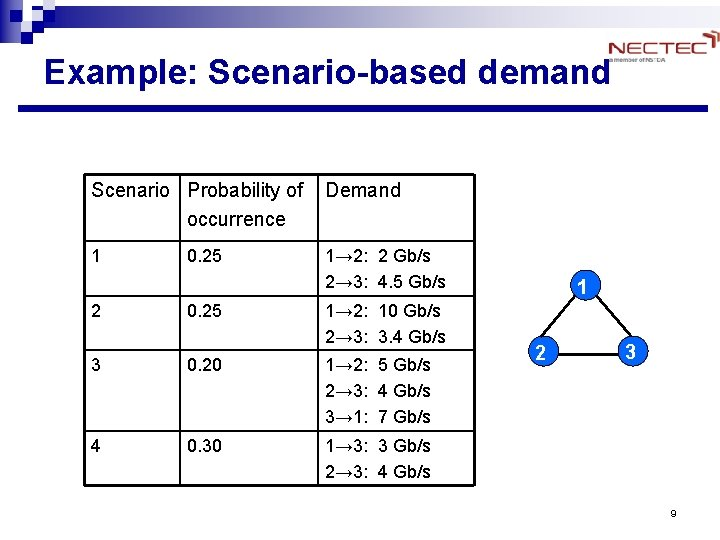Example: Scenario-based demand Scenario Probability of occurrence Demand 1 1→ 2: 2 Gb/s 2→ 3: 4. 5 Gb/s 0. 25 2 0. 25 1→ 2: 10 Gb/s 2→ 3: 3. 4 Gb/s 3 0. 20 1→ 2: 5 Gb/s 2→ 3: 4 Gb/s 3→ 1: 7 Gb/s 4 0. 30 1→ 3: 3 Gb/s 2→ 3: 4 Gb/s 1 2 3 9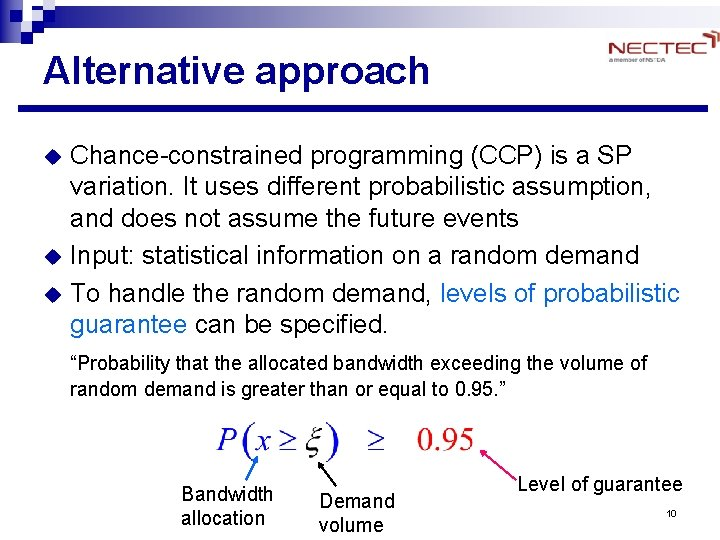Alternative approach u u u Chance-constrained programming (CCP) is a SP variation. It uses different probabilistic assumption, and does not assume the future events Input: statistical information on a random demand To handle the random demand, levels of probabilistic guarantee can be specified. “Probability that the allocated bandwidth exceeding the volume of random demand is greater than or equal to 0. 95. ” Bandwidth allocation Demand volume Level of guarantee 10CCP Medova  studies routing and link bandwidth allocation problem in an ATM network u Ø Ø Level of guarantee = 1 - Probability of blocking ATM connection request Assumes that this Prob. is very small the approximation eliminates statistical information of random demands. Our work u Ø Ø Levels of guarantee are used. Each demand has its own guarantee value. Aggregate traffic carried on each link is composed of two parts: certain and uncertain parts. 11CCP Ø Demand statistics l A random variable x has mean (m ) and variance ( s 2 ) Ø Probabilistic guarantee ( ai ) Ø The amount of bandwidth to be allocated, x 12Example: CCP Formulation Minimize total bandwidth cost Subject to: (3. 2) Chance constraints -guarantee a random demand with some level (3. 3) (3. 4) Bandwidth constraints -set limitation on network resources Non-negativity constraints 13Deterministic Equivalent u Deterministic equivalent of stochastic constraint x is N (m, s 2) (. ) = cumulative distribution function -1(. ) = inverse transform of (. ) “certain factor” “uncertain factor” To guarantee that the link can support the random demand at least a-level, we need to allocate bandwidth at least -1(a)s beyond the mean m of the demand volume. 14Multiple demands Each flow is guaranteed with a-level. E. g. Let a = 0. 95, -1(0. 95) = 1. 645 Random variables: x 1, x 2, …, x 10 mk = 100, s 2 = 100 Sum-part 1 = 100*10 = 1000 Sum-part 2 =1. 645*(10*10) = 164. 5 15Proposed research u Goal Ø Ø u To develop a methodology for network design under demand uncertainty. Need to solve a routing and bandwidth allocation problem based on CCP so achieve the benefits from statistical guarantee. Research Approach Ø Ø To develop mathematical models for a routing and bandwidth allocation problem with uncertainty constraints. This is intended for usage in the IP layer, and will not solve the traffic glooming problem in the physical layer. 16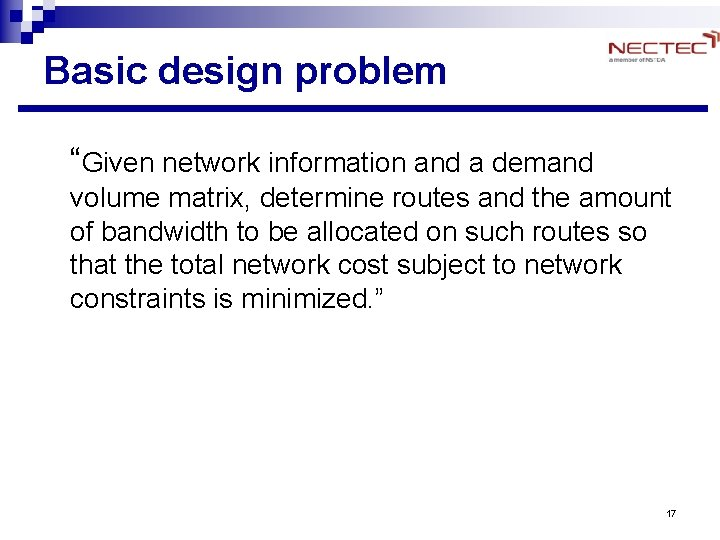Basic design problem “Given network information and a demand volume matrix, determine routes and the amount of bandwidth to be allocated on such routes so that the total network cost subject to network constraints is minimized. ” 17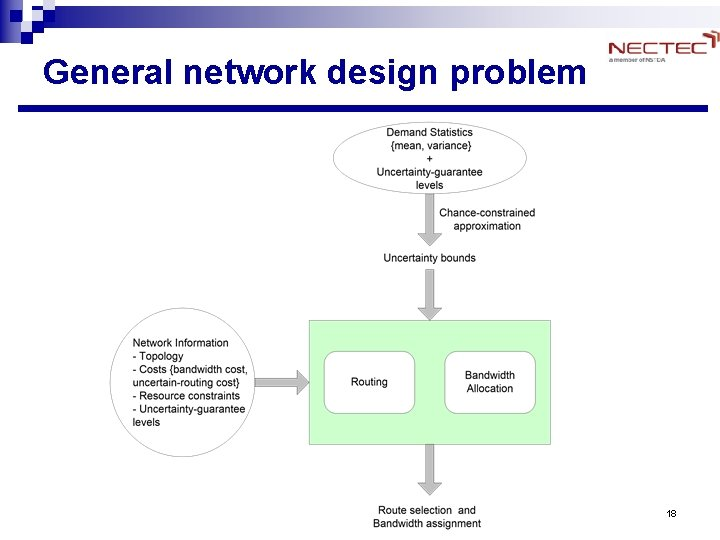General network design problem 18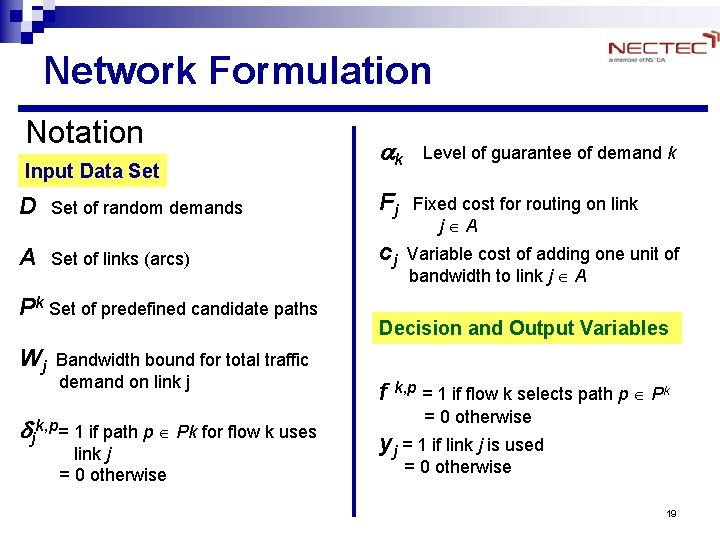Network Formulation Notation Input Data Set ak Level of guarantee of demand k D Set of random demands Fj Fixed cost for routing on link A Set of links (arcs) cj Variable cost of adding one unit of Pk Set of predefined candidate paths j A bandwidth to link j A Decision and Output Variables Wj Bandwidth bound for total traffic demand on link j f k, p = 1 if flow k selects path p Pk d = 0 otherwise k, p j = 1 if path p Pk for flow k uses link j = 0 otherwise yj = 1 if link j is used = 0 otherwise 19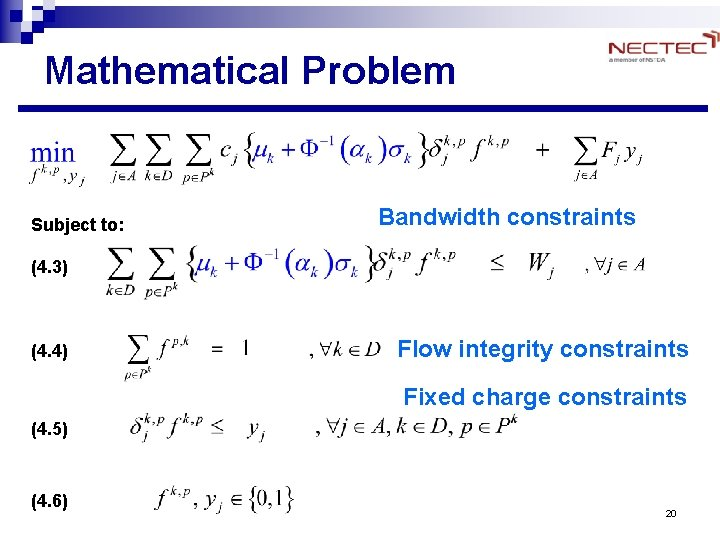Mathematical Problem Subject to: Bandwidth constraints (4. 3) (4. 4) Flow integrity constraints Fixed charge constraints (4. 5) (4. 6) 20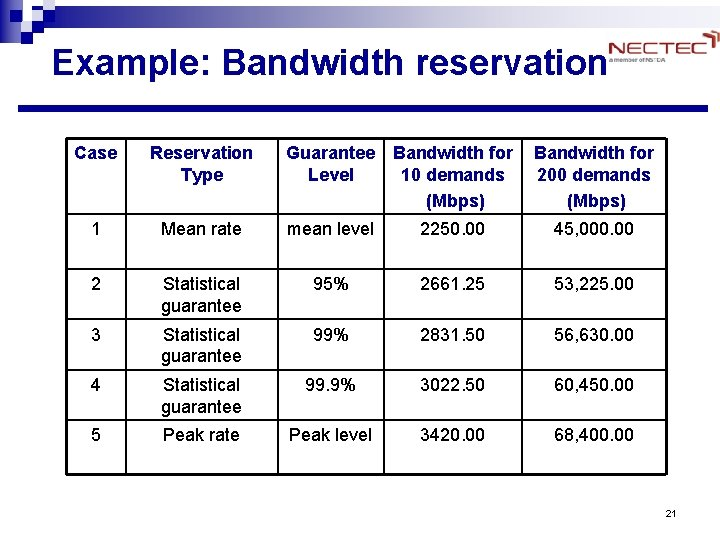Example: Bandwidth reservation Case Reservation Type Guarantee Bandwidth for Level 10 demands (Mbps) Bandwidth for 200 demands (Mbps) 1 Mean rate mean level 2250. 00 45, 000. 00 2 Statistical guarantee 95% 2661. 25 53, 225. 00 3 Statistical guarantee 99% 2831. 50 56, 630. 00 4 Statistical guarantee 99. 9% 3022. 50 60, 450. 00 5 Peak rate Peak level 3420. 00 68, 400. 00 21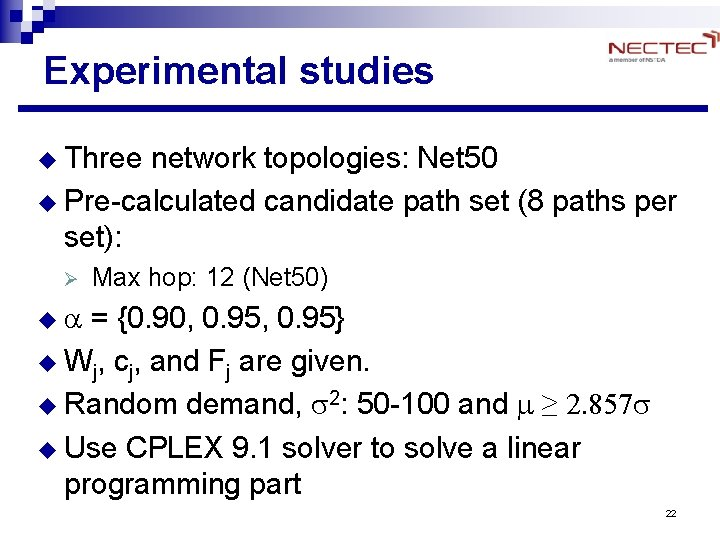Experimental studies u Three network topologies: Net 50 u Pre-calculated candidate path set (8 paths per set): Ø Max hop: 12 (Net 50) u a = {0. 90, 0. 95} u Wj, cj, and Fj are given. u Random demand, s 2: 50 -100 and m ≥ 2. 857 s u Use CPLEX 9. 1 solver to solve a linear programming part 22Net 50 (50 nodes, 82 links) 23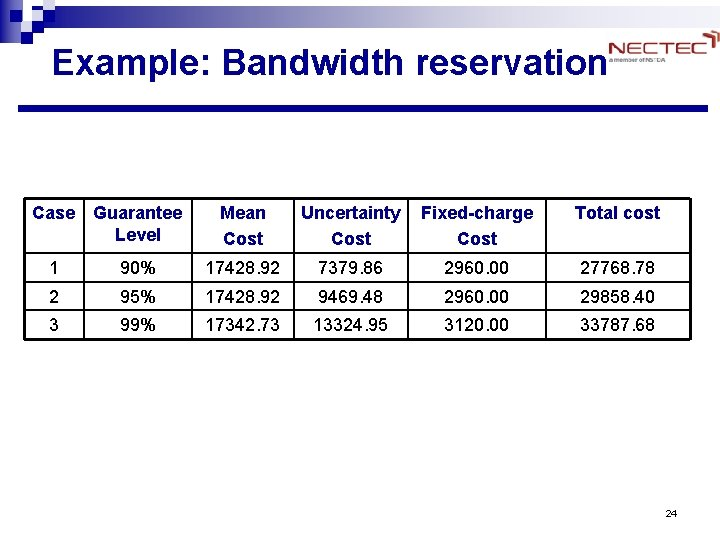Example: Bandwidth reservation Case Guarantee Level Mean Cost Uncertainty Cost Fixed-charge Cost Total cost 1 90% 17428. 92 7379. 86 2960. 00 27768. 78 2 95% 17428. 92 9469. 48 2960. 00 29858. 40 3 99% 17342. 73 13324. 95 3120. 00 33787. 68 24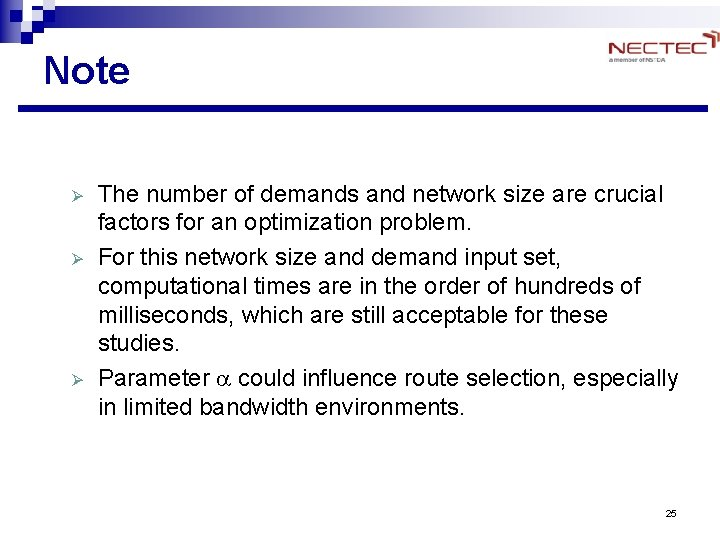Note Ø Ø Ø The number of demands and network size are crucial factors for an optimization problem. For this network size and demand input set, computational times are in the order of hundreds of milliseconds, which are still acceptable for these studies. Parameter a could influence route selection, especially in limited bandwidth environments. 25Conclusions u Theoretical study 1. A new interpretation of the Chance. Constrained Programming optimization in the communications networks context, considering both the uncertainty and service guarantees. 2. A mathematical formulation for network design under traffic uncertainty is developed. This framework is expected to be applied to the virtual network design at the IP layer. l l The uncertainty model is based on short-term routing and bandwidth provisioning. Uses Chance-constraints to capture both the demand variability and levels of uncertainty guarantee. 26Conclusions Future work: improvement of accuracy of the model u Ø Ø Ø Simulation studies on the relation between different traffic patterns and the benefit of the Chanceconstraint approximation are needed. Traffic measurement: An investigation on other traffic distributions and their effects on the uncertainty bound. A study on the benefits of the scheme with real traffic input from measurement. 27Questions? Thank You 28